# Optimization and parallelization of tensor and ODE/PDE computations on GPU

N/A
N/A
Protected

Share "Optimization and parallelization of tensor and ODE/PDE computations on GPU"

Copied!
46
0
0

Full text

We propose a multilevel GPU-based parallelization algorithm to solve the multipartition Hodgkin Huxley (HH) model equation that requires solving the Hines matrix. Our approach uses CUDA's dynamic parallelism to achieve multi-level parallelism on the GPU. The main goal of this work was to try to derive multilevel parallelism for solving the Hodgkin Huxley set of differential equations, which are used to model the action potential of a neuron.

This work uses two separate parallelization algorithms and combines them to achieve multi-level parallelism to solve the set of equations and the results are obtained on a GPU.

## Optimizing Higher Order Tensor Renormalization Group

The first and second problems are optimization problems where the goal is to make the calculation faster, and this was done by implementing on GPU and taking advantage of its parallelism. The third problem was an implementation job that takes an outdated open source tool and updates it to the latest version.

## Legup: High Level Synthesis with LLVM

In solving time-dependent systems of ordinary/partial differential equations using implicit methods, the given system of linear equations must be solved once for each time step. However, when solving systems of differential equations, these ideas only help speed up the time taken for a single time step. When solving for a large number of time steps, each time step requires the solutions of the previous time step.

Previous work on the parallel solution of the HH equation has focused only on solving the linear system that occurs at each time step.

## Tridiagonal and Hines Matrix

### Tridiagonal Matrix

When implicit finite difference methods are applied to the PDEs derived from such models, the resulting equation requires the solution of a system of linear equations where the coefficient matrix has a sparse structure. For example, applying the backward Euler method to the above cable equation 2.1 leads to the equation. With the cable equation for a linear dendritic model, as shown in equation 2.1, we obtain a tridiagonal matrix which must be solved at each time step.

One of the famous algorithms for solving a tridiagonal system of equations is TDMA (Tridiagonal Matrix Algorithm), also known as Thomas' Algorithm by Thomas et al.

### Hines Matrix

The rearrangement of this equation provides us with a system of linear equations where the coefficient matrix is ​​formed by the tridiagonal matrix Vi+1, Vi, and Vi−1, where Vi represents the voltage of the i-th division of the neuron. Hines' idea was to count the nodes of the tree in a deep way, so that the child always counted less than the parent. With this ordering, we can construct the tree adjacency matrix where each row depends only on its lower rows.

The adjacency matrix of the tree constructed in Figure 1.2 yields the Hines matrix, shown in Figure 2.3.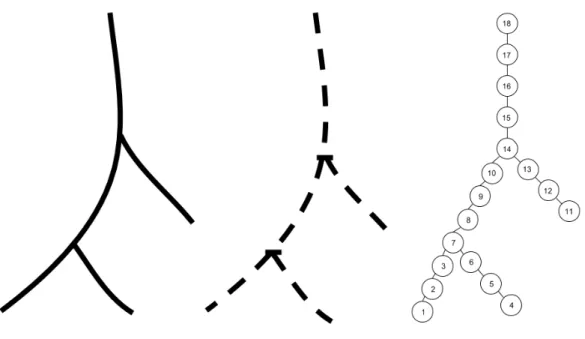Figure 2.2: Example of compartmentalizing the neuron and visualizing it as a tree. The tree has been numbered in depth first order

## Related Work

• Background on Neuroscience
• Prior work on solving Hines matrix
• Prior work on Temporal Discretization
• Contributions

It then calculates the final solution using solutions for these smaller matrices and solutions for a “domain” matrix. Roy Ben-Shalom et al. proposed a way to solve the system using GPUs to accelerate the compartmental modeling. In general, the parallel space-time algorithms can be classified into four categories depending on how the parallel solver strategy is applied.

These are methods based on multigrid, direct time-parallel methods, multiple shooting and methods based on domain decomposition and waveform relaxation. The first work in the parallel algorithm over time domain was by Nievergelt et al. These methods are not parallel by default, but their parts can be executed in parallel space-time domain since simultaneous work is done on the entire space-time domain.

21] in 2010 with the goal of creating parallel time integration algorithms suitable for multi-core architectures, resulting in small-scale parallelism. The main contribution of this work is the use of parallelism to solve the Hodgkin-Huxley equation. To exploit the parallelism at the internal level, where the solution of a linear system with the Hines matrix is ​​required, we use Mascagni's algorithm, which is based on the exact decomposition of the domain .

Parareal Algorithm

## Hines Matrix Solver using EDD

So if we can find the node xjunction for each junction, we can replace this with findxi. The junction node values ​​are found by substituting the x0i and x1i values ​​into the equation containing the junction node, giving the system only the junction node values ​​as unknowns.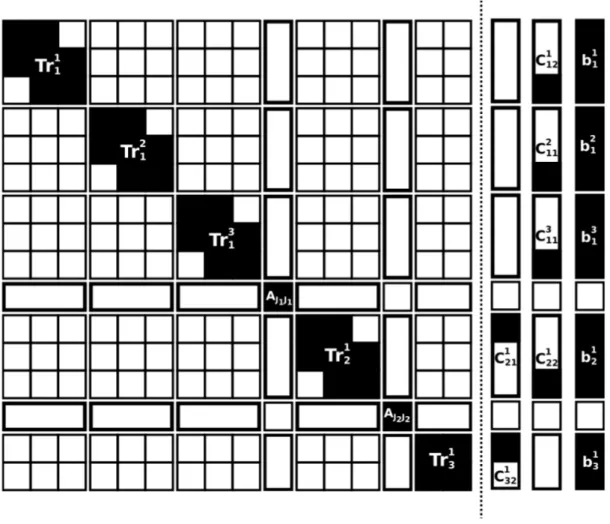Figure 2.5: Example of EDD split on hines matrix and the corresponding 3 RHS values

Combining Parareal and EDD algorithm

## Experimental setup and implementation details

### Matrix storage details

The D vector stores the main diagonal elements and the U vector stores the only non-zero element that exists after the main diagonal.

## Results

As commonly known in the literature and shown in the graph below, when the size of the Hines matrix is ​​relatively small (i.e. less than 1000), it is better to run the algorithm on a CPU, but when the size of the Hines matrix is ​​large, the speedup compared to the CPU is quite good .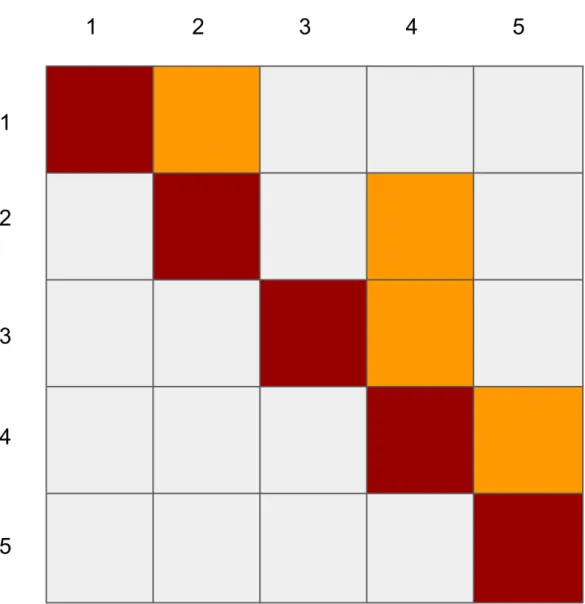Figure 2.7: Shows the D and U vector stored. Since Hines matrix is symmetric, the lower triangular values need not be stored.

## Future Work

The differential equations used in IF models are relatively much simpler and can be solved analytically. To capture a realistic action of neurons in a neural network, the Action Potential must be modeled, which can be done with the Hodgkin-Huxley model. The problem of considering Hodgkin-Huxley for a network of neurons is therefore a much more important problem, but due to the high number of neurons in the brain and the numerical solutions required for the HH-based differential equations, this problem computationally much more expensive.

So, trying to use the methods in this work to help model a network models using HH model will be a much more important and interesting work.

## Conclusion

Tensor calculations are used in many scientific problems in quantum physics, quantum chemistry and many other fields. Some of the main tensor calculations are tensor addition, tensor contraction, cross product, etc. This section discusses the optimization and parallelization challenges for a tensor contraction-based algorithm that attempts to compute emergent phenomena in many-body quantum systems.

The big picture of the problem being solved is given by a bunch of electrons, atoms, etc. This work provided an initial tensor renormalization group algorithm that generalized the density matrix renormalization group (the TRG algorithm to two-dimensional classical mesh models using singular value decomposition 34] where they improved the accuracy of the original TRG algorithm, called the Second Renormalization Group (SRG).

Mathematically, the algorithm approximates a tensor that has a large rank with a network of tensors of smaller ranks. From a computational point of view, the problem to be solved is to optimize memory and computational resource usage when computing tensor contraction for high-dimensional tensors.

## Tensor computations

### Tensor networks

They are indicated by connecting two dots (representing the tensors) with a common leg as shown in figure 4.1.

Operations on Tensors

## Initial Optimizations and results

In this particular algorithm, the tensor contraction is between two tensors, one of high dimension and another of lower dimension, but they share only one index. If the values ​​in the tensor are stored in order of magnitude, this computation would access non-contiguous elements of the array, which would cause cache misses for each element of the main array that stores the tensor. An important way to avoid cache misses is to swap the loops so that the array accesses for the tensor in one of the arrays are contiguous to avoid cache misses.

The problem with loop exchange is that if there are dependencies across accesses in the array, the dependencies must be maintained in the exchanged code. We used the polyhedral optimization tool pluto to optimize the code, but the cost model of the tool did not concentrate on the loop exchange. In the graph above, the names below the bars represent the function names that contain the calculations.

The getu* functions contain the rearrangement of values, and gett* functions contain the Tensor contraction.

## GPU Optimization

The above shows the percentage speedup between a single GPU and a multi-GPU version of the code. This is because the overhead of splitting the matrices to be computed into multiple GPUs and the communication overhead exceeds the gain.

## Exact Computation and possible solutions using TCE

Since we don't need the values ​​of the intermediate tensors in full, if we can compute T1 and T2 partially, this could be used to compute the corresponding values ​​of A. Removing the intermediate tensors would save loop pooling time and memory because the 2 intermediate tensors of rank 8 never need to be fully preserved in memory. This would provide significant savings, since if the value of D is 16, then the number of elements in a rank 8 tensor whose dimension size is D is 168.

Thus, the total memory required to store a single rank-8 tensor of size D=16 is 168*8 bytes, which is equal to 32 GB.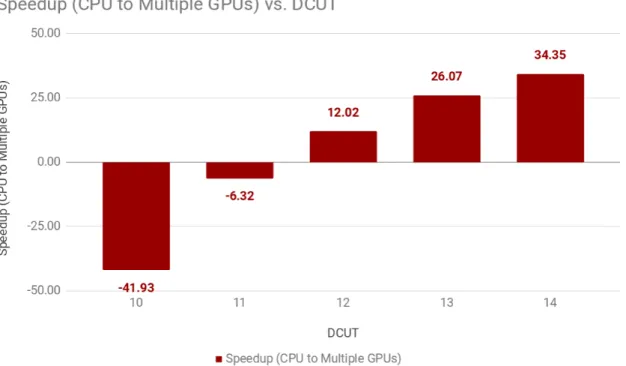Figure 3.12: Speedup between single and multiple GPU exact sequence of contractions are shown below:

## Conclusion

Programming in HDL/RTL type languages ​​was easy in the beginning, but as devices became more complex, the requirements of encryption algorithms, deep learning, etc.

## High Level Synthesis

### Scheduling

Planning is the process of dividing the control flow of the algorithm into parts that can be used to define the states of a finite state machine. The finite state machine is used to define the steps of the computation, which are then synthesized. Each part/step is used to execute a small step of the algorithm, which can be executed in a single clock cycle.

Allocation

Binding

Related Tools

Basic architecture of the tool

## My work

In addition to these code changes, the Legupa verilog backend depends on configuration files read in as TCL scripts that contain hardware configurations for specific FPGA hardware. After the changes, we built and tested the verilog backend with the test cases provided as part of the Legup source code. An optimized Schwarz waveform relaxation method for the unsteady convective diffusion equation in two dimensions.

Splitting neurons in compute-bound parallel network simulations enables runtime scaling with twice as many processors. A time-parallel implicit method for accelerating the solution of nonlinear structural dynamics problems. International Journal for Numerical Methods in Engineering. In Proceedings of the International Conference on High Performance Computing, Networking, Storage and Analysis, SC.

Figure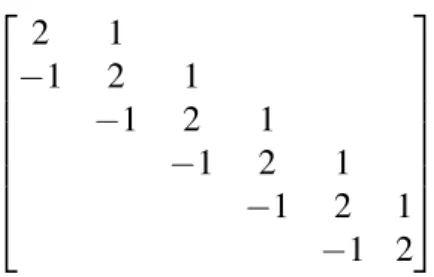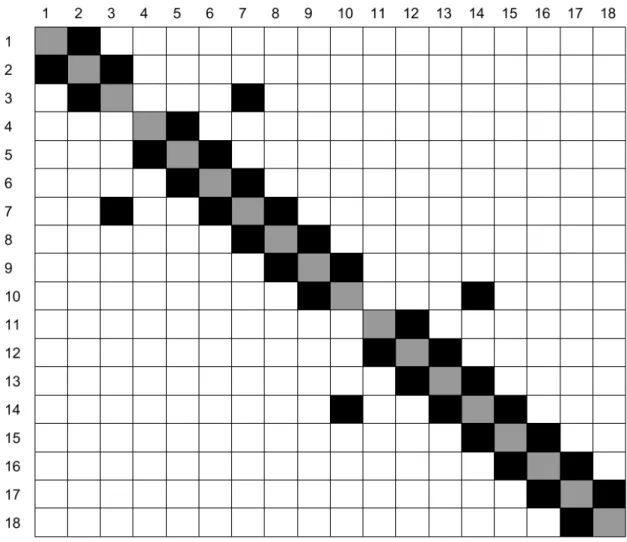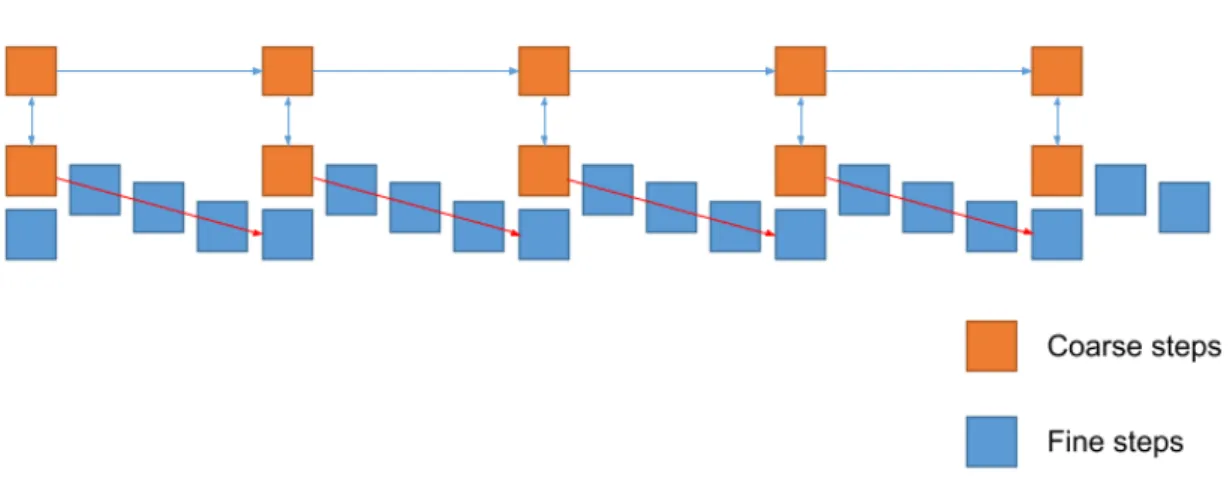+7

References

Outline

Related documents

To conﬁrm that the same results can be obtained even when the wasps have a choice, we offered a choice of two virgin partners either to a virgin test male or to a virgin test female and

The MCGL method is able to model the decision making process of individuals under the framework of MCDA, by revising some of the MCDM mainstream postulates and practices in order to

In the process of regularly taking care of plants and animals and tending to them, the educator forms certain work skills in children, teaches them to pay attention to the animals in

The vision of the National Education Policy is: Na- tional Education Policy 2020 envisions an India- centric education system that contributes directly to transforming our nation

The purpose of this high level conference was to assess the accomplishments and shortcomings of the Guiding Principles on Internal Displacement over the last 10 years, and to chart a

Since almost 93 per cent of the work force in India is in the informal sector with virtually no rights, and almost half of them are Dalits and women, this study will focus on Dalits,

The candidates will write the correct Test Booklet Code and Number as given in the Test Booklet / Answer Sheet in the Attendance Sheet.. A machine will read the coded information in the

The main objectives of the present work are i to study the charging and discharging characteristics of a LHS prototype by considering the natural convection effect, ii to develop a 2D

• Are the proposed multi-context model and the individual single-context models able to separate the examples from the different classes?To understand whether the representa- tions

To this effect, a supervised selection of features is proposed using a neural network to achieve better or comparable classification performance.. Runtime gains are also obtained as the